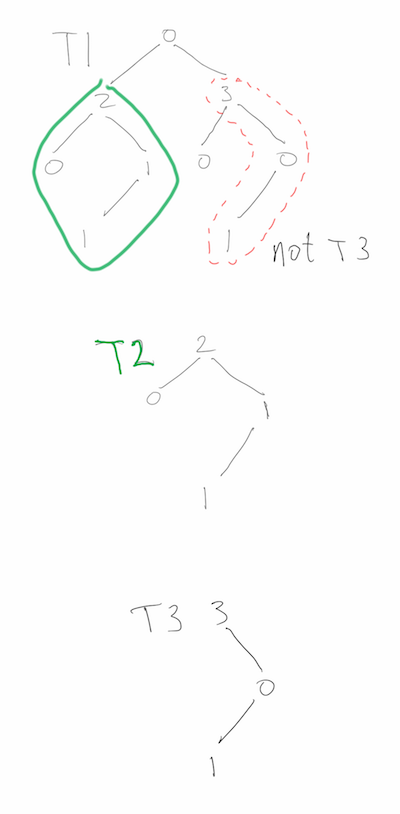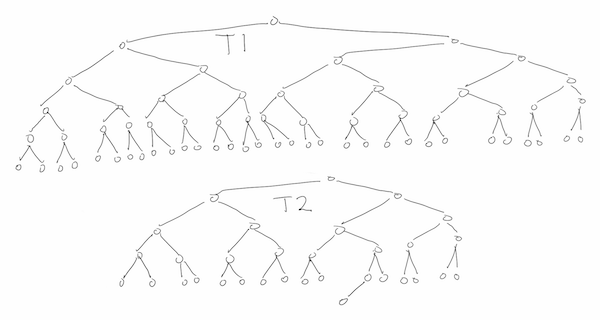“Cracking the Coding Interview 6e” question 4.10: T1 and T2 are binary trees with m and n nodes, respectively. Test whether T1 contains T2 as a subtree. Here is my solution, which performs much better than the book’s solution.

The problem asks, more specifically, if there is a node in T1 such that the subtree rooted there has exactly the same structure as T2, as well as the same values at each node. Spoilers follow the image below, so if you are looking for hints, proceed carefully.Test subtree containment

CCtI6e discusses two solutions, the first one makes the observation that a tree is uniquely identified by its pre-order traversal if you include special identifiers for null child-nodes, so the tree containment problem is reduced to a substring problem: does toString(T1) contain toString(T2)? CCTI6e blurs the solution by using a library call to solve the substring problem (it is not trivial to do it efficiently), but they do claim that this solution runs in O(m+n) time and O(m+n) space. It’s a valid answer, but I want something better.

The second solution tries to improve on the O(m+n) space of the first solution. The naïve way would be to do a depth-first search comparison of T2 and the subtree rooted at each node of T1, resulting in an O(log(m) + log(n)) space algorithm that uses O(min(m*log(m),m*n)) time. CCtI6e suggests using the values of the root of the T1-subtree and the root of T2 to decide whether or not to perform the search, but this ultimately makes no difference at all. First, if the values are different, then the search would abort immediately, and second, the nodes of T1 and T2 could have all the same data, say 0. Below is a drawing of a bad case, where most of T2 occurs at many nodes of T1, but T1 does not contain T2.A bad case for recursive subtree testing

I was surprised to see such naïve solution to this problem, as CCtI6e has been very good so far. The recursive solution can be improved upon considerably by using a better heuristic for whether or not to search/compare T2 with the subtree at a given node of T1.

### Hash the structure and data of a binary tree

We want to hash each node so that if the hashes of two trees match, there is a high probability that their subtrees match. As a proof of concept, I adapted Knuth’s multiplicative method to work on binary trees with integer values. The idea is to re-hash the hash values of the left and right children, as well as the height and value of the current node, using distinct 32-bit primes for each part, and then add the four multiplicative hash values. I’ve read that hashing is part guess-work and part theory, and there you have it.

uint32_t hash(uint64_t left,
uint64_t right,
uint64_t value,
uint64_t height){
return ((left&(0xFFFFFFFF))*2654435761 +
(right&(0xFFFFFFFF))*2654435741 +
(value&(0xFFFFFFFF))*2654435723 +
(height&(0xFFFFFFFF))*2654435713);
}

uint32_t dfs_hash(node *t){
if(!t) return 0;
return hash(dfs_hash(t->left),dfs_hash(t->right),t->value,t->height);
}


My hash function seems to work quite well for randomly generated trees, but it is not thoroughly tested or anything. I’d like to eventually adapt a more sophisticated function, perhaps from Eternally Confuzzled.

### Using the tree-hashes

My algorithm works as follows:

• pre-compute the hash of T2.
• Do a depth-first search of T1 where at each node:
• if one of child nodes of the current node contains T2, return True. Otherwise, record the hashes of the child nodes.
• hash the current node in O(1) time
• if the hash of the current node matches the hash of T2:
• do a depth-first search that compares T2 to the subtree rooted at the current node
• if T2 is identical to the subtree rooted at the current node, then return True
• return the hash of the current node.

Note that I do not cache hash values. The idea in this exercise is not to use unnecessary space, beyond the O(log(m)+log(n)) space necessarily used by depth-first searches. Caching hash values would not only use O(n+m) additional space, but keeping the hash values up to date in a non-static binary tree requires modifying the binary tree implementation.

The above pseudo-code is implemented below, and the complete source-code is at the end of this blog post.

typedef struct node{
int value,height;
struct node *left;
struct node *right;
}node;

node *rec_contains_subtree(node *t1, node *t2, uint32_t *t1ChildHash, uint32_t t2hash){
uint32_t leftChildHash,rightChildHash;
node *res;
*t1ChildHash=0;
if(!t1)
return NULL;
if((res=rec_contains_subtree(t1->left,t2,&leftChildHash, t2hash))||
(res=rec_contains_subtree(t1->right,t2,&rightChildHash,t2hash)))
return res;
count_nodes_hashed++;
*t1ChildHash=hash(leftChildHash,rightChildHash,t1->value,t1->height);
if(*t1ChildHash==t2hash){
count_dfs_compare++;
if(dfs_compare_trees(t1,t2))
return t1;
}
return NULL;
}

node *contains_subtree(node *t1, node *t2){
//hash t2
uint32_t t2hash = dfs_hash(t2);
uint32_t t1ChildHash;
if(!t2)
exit(-1);
return rec_contains_subtree(t1,t2,&t1ChildHash,t2hash);
}


Below is the other depth-first search, that compares T2 with a subtree of T1. Notice that the CCtI6e solution is implicit in the last conditional.

int dfs_compare_trees(node *t1, node *t2){
if(!t1 && !t2)
return 1;
if(!t1 && t2)
return 0;
if(t1 && !t2)
return 0;
if(t1->value!= t2->value)
return 0;
return dfs_compare_trees(t1->left,t2->left) &&
dfs_compare_trees(t1->right,t2->right);
}


### Testing on some large inputs

I did some tests on randomly generated trees. This is by no means a robust test, especially as regards hash collisions. I’ll paste some output and then explain it.

Below, I insert 10,000,000 nodes into T1 and 100 nodes into T1, by descending the trees on randomly selected branches and inserting at the first empty node. All data values are 0s. Since the result is that T1 doesn’t contain T2, then all 10,000,000 nodes of T1 are searched, but notice that the hashing saves me from doing any node-by-node comparisons with T2.

1e-05 seconds to allocate trees
10.3298 seconds to create t1
2e-05 seconds to create t2
t1 has 10000000 nodes
t2 has 100 nodes
0.928297 seconds to check if t1 contains t2
t1 does not contain t2
Did dfs_compare_trees 0 times
Hashed 10000000 nodes of t1


With the same tree T1 as above, I regenerated T2 100 times and repeated the same test as above. Again, the node-by-node comparison, dfs compares, was done 0 times (per search).

Test containment in t1 of randomly generated trees t2
Nodes: t1 10000000 t2 100; Trials: 100
All nodes hold value=0
Average per search:
time 0.944213;
dfs compares 0;
prop. nodes hashed 1;
containment 0


I repeated the previous experiment, below, with smaller trees T2, on 20 nodes. Now we see that 6/100 of these T2s are contained in T1, but dfs_compares is done 10 times more often than we find something. It shows that the hashing is giving quite a few false positives for small T2! More testing needed.

Test containment in t1 of randomly generated trees t2
Nodes: t1 10000000 t2 20; Trials: 100
All nodes hold value=0
Average per search:
time 0.96471;
dfs compares 0.58;
prop. nodes hashed 0.964319;
containment 0.06


In order to test containment of larger trees T2, I simply used subtrees of T1, selected (pseudo) uniformly at random. Since my search does not consider the values of the references to the nodes, it is unaware that T1 and T2 share locations in memory. Below we see that very few nodes of T1 are hashed, and that a hash collision is experienced in about 12% of the searches, since 1.12=dfs_compares/containment.

Test containment in t1 of randomly selected subtrees of t1
Nodes: t1 10000000 t2 (unknown); Trials: 100
All nodes hold value=0
Average per search:
time 0.0682518;
dfs compares 1.12;
prop. nodes hashed 0.0711984;
containment 1


The running time is difficult to compute (and maybe impossible to prove), because of the way it depends on the hash function. Informally, we can state an expected time of O(m+(1+c)n), where c is the number of hash collisions. If c is small enough, this becomes O(m). This is much better than CCtI6e’s time of O(m+kn), where k is the number of nodes of T1 that have the same value as the root of T2, since, in our 0-value trees, that could easily amount to O(m*log(m)); the cost of doing a full depth-first search of T1 at every node.

The code, which is a bit rough and quick, is available as a gist: